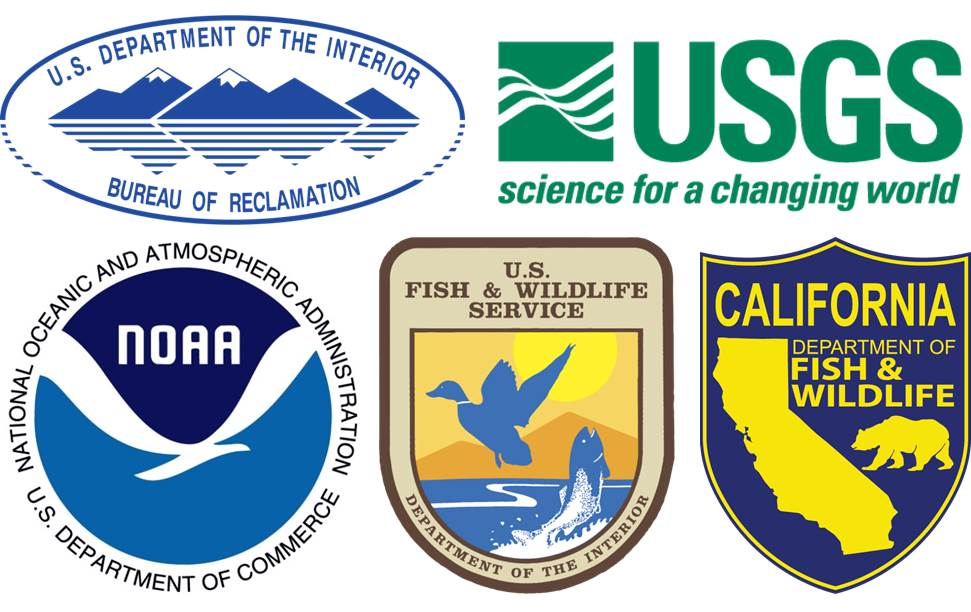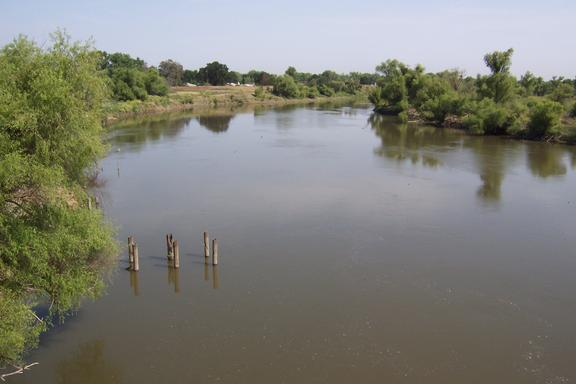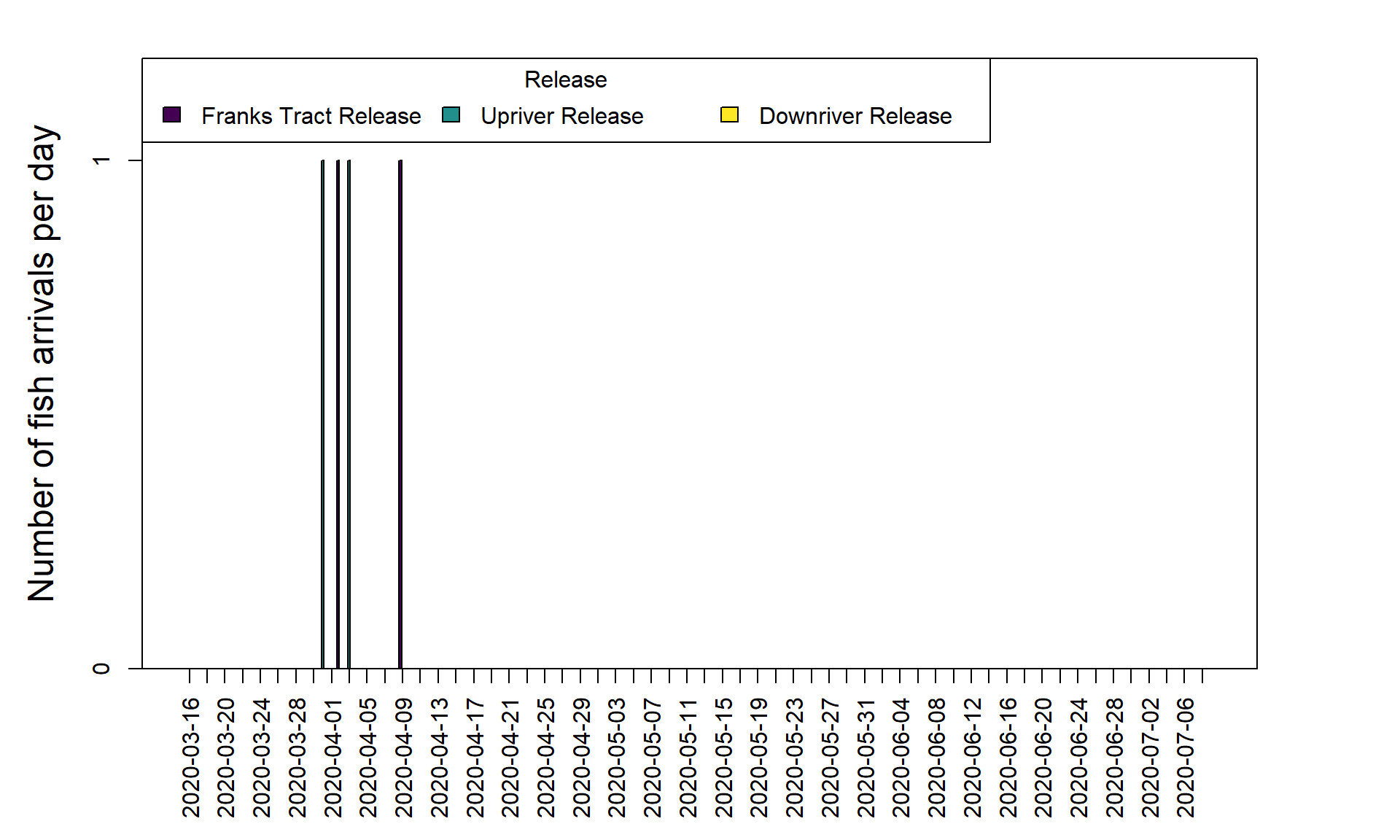# Acoustic Tagging Project# Hatchery-origin San Joaquin spring-run Chinook salmon

## 1. Project Status

Telemetry Study Template for this study can be found here

setwd(paste(file.path(Sys.getenv("USERPROFILE"),"Desktop",fsep="\\"), "\\Real-time data massaging\\products", sep = ""))

tagcodes <- as.data.frame(fread("qry_HexCodes.txt", stringsAsFactors = F))
tagcodes$RelDT <- as.POSIXct(tagcodes$RelDT, format = "%m/%d/%Y %I:%M:%S %p", tz = "Etc/GMT+8")

study_tagcodes <- tagcodes[tagcodes$StudyID == "SCARF_San_Joaquin_Spring_run_2020",] if (nrow(study_tagcodes) == 0){ cat("Project has not yet begun") }else{ cat(paste("Project began on ", min(study_tagcodes$RelDT), ", see tagging details below:", sep = ""))

study_tagcodes$Release <- "Upriver Release" study_tagcodes[study_tagcodes$Rel_loc == "Durham_Ferry", "Release"] <- "Downriver Release"
study_tagcodes[study_tagcodes$Rel_loc == "Franks_Tract", "Release"] <- "Franks Tract Release" #study_tagcodes[study_tagcodes$RelDT > as.POSIXct("2020-03-20"), "Release"] <- "Release 2"

release_stats <- aggregate(list(First_release_time = study_tagcodes$RelDT), by= list(Release = study_tagcodes$Release),
FUN = min)
release_stats <- merge(release_stats,
aggregate(list(Last_release_time = study_tagcodes$RelDT), by= list(Release = study_tagcodes$Release),
FUN = max),
by = c("Release"))

release_stats <- merge(release_stats, aggregate(list(Number_fish_released =
study_tagcodes$TagID_Hex), by= list(Release = study_tagcodes$Release),
FUN = function(x) {length(unique(x))}),
by = c("Release"))

release_stats <- merge(release_stats,
aggregate(list(Release_location = study_tagcodes$Rel_loc), by= list(Release = study_tagcodes$Release),
by = c("Release"))
release_stats <- merge(release_stats,
aggregate(list(Release_rkm = study_tagcodes$Rel_rkm), by= list(Release = study_tagcodes$Release),
by = c("Release"))
release_stats <- merge(release_stats,
aggregate(list(Mean_length = study_tagcodes$Length), by= list(Release = study_tagcodes$Release),
FUN = mean, na.rm = T),
by = c("Release"))
release_stats <- merge(release_stats,
aggregate(list(Mean_weight = study_tagcodes$Weight), by= list(Release = study_tagcodes$Release),
FUN = mean, na.rm = T),
by = c("Release"))

release_stats2<-release_stats[,-3]
colnames(release_stats2)<-"Release time"

release_stats[,c("Mean_length", "Mean_weight")] <- round(release_stats[,c("Mean_length", "Mean_weight")],1)

release_stats$First_release_time <- format(release_stats$First_release_time, tz = "Etc/GMT+8")

release_stats$Last_release_time <- format(release_stats$Last_release_time, tz = "Etc/GMT+8")

kable(release_stats, format = "html") %>%
kable_styling(bootstrap_options = c("striped", "hover", "condensed", "responsive", "bordered"), full_width = F, position = "left")
}                       
## Project began on 2020-03-16 19:30:00, see tagging details below:
Release First_release_time Last_release_time Number_fish_released Release_location Release_rkm Mean_length Mean_weight
Downriver Release 2020-03-24 23:30:00 2020-03-24 23:30:00 350 Durham_Ferry 181.80 81.6 5.8
Franks Tract Release 2020-03-24 21:41:00 2020-03-24 21:41:00 100 Franks_Tract 101.74 81.5 5.7
Upriver Release 2020-03-16 19:30:00 2020-03-16 19:30:00 350 Fremont_Ford 270.93 80.9 5.8

## 2. Real-time Fish Detections

Study is complete, tags are no longer active. All times in Pacific Standard Time.

setwd(paste(file.path(Sys.getenv("USERPROFILE"),"Desktop",fsep="\\"), "\\Real-time data massaging\\products", sep = ""))

library(CDECRetrieve)
library(reshape2)

detects_study <- fread(paste(file.path(Sys.getenv("USERPROFILE"),"Desktop",fsep="\\"), "\\Real-time data massaging\\products\\Study_detection_files\\detects_SCARF_San_Joaquin_Spring_run_2020.csv", sep = ""))

if(nrow(detects_study)>0){
detects_study$DateTime_PST <- as.POSIXct(detects_study$DateTime_PST, format = "%Y-%m-%d %H:%M:%S", "Etc/GMT+8")
detects_study <- merge(detects_study, study_tagcodes[,c("TagID_Hex", "RelDT", "StudyID", "Release", "tag_life")], by.x = "TagCode", by.y = "TagID_Hex")
}

detects_benicia <- detects_study[detects_study$general_location %in% c("Benicia_west", "Benicia_east"),] if (nrow(detects_benicia)>0) { detects_benicia <- merge(detects_benicia,aggregate(list(first_detect = detects_benicia$DateTime_PST), by = list(TagCode= detects_benicia$TagCode), FUN = min)) detects_benicia$Day <- as.Date(detects_benicia$first_detect, "Etc/GMT+8") starttime <- as.Date(min(detects_benicia$RelDT), "Etc/GMT+8")
#endtime <- as.Date(c(Sys.time()))#, max(detects_benicia$first_detect)+60*60*24))) #wlk_flow <- cdec_query("COL", "20", "H", starttime, endtime+1) #wlk_flow$datetime <- as.Date(wlk_flow$datetime) #wlk_flow_day <- aggregate(list(parameter_value = wlk_flow$parameter_value), by = list(Day = wlk_flow$datetime), FUN = mean, na.rm = T) endtime <- min(as.Date(format(Sys.time(), "%Y-%m-%d")), max(as.Date(detects_benicia$RelDT)+(detects_benicia$tag_life*1.5))) daterange <- data.frame(Day = seq.Date(from = starttime, to = endtime, by = "day")) rels <- unique(study_tagcodes[study_tagcodes$StudyID == unique(detects_benicia$StudyID), "Release"]) rel_num <- length(rels) rels_no_detects <- as.character(rels[!(rels %in% unique(detects_benicia$Release))])

tagcount <- aggregate(list(unique_tags = detects_benicia$TagCode), by = list(Day = detects_benicia$Day, Release = detects_benicia$Release ), FUN = function(x){length(unique(x))}) tagcount1 <- dcast(tagcount, Day ~ Release) daterange1 <- merge(daterange, tagcount1, all.x=T) if(length(rels_no_detects)>0){ for(i in rels_no_detects){ daterange1 <- cbind(daterange1, x=NA) names(daterange1)[names(daterange1) == 'x'] <- paste(i) } } #daterange2 <- merge(daterange1, wlk_flow_day, by = "Day", all.x = T) daterange2 <- daterange1 rownames(daterange2) <- daterange2$Day
daterange2$Day <- NULL par(mar=c(6, 5, 2, 5) + 0.1) barp <- barplot(t(daterange2[,1:ncol(daterange2)]), plot = FALSE, beside = T) barplot(t(daterange2[,1:ncol(daterange2)]), beside = T, col=viridis_pal()(rel_num), xlab = "", ylab = "Number of fish arrivals per day", ylim = c(0,max(daterange2[,1:ncol(daterange2)], na.rm = T)*1.2), las = 2, xlim=c(0,max(barp)+1), cex.lab = 1.5, yaxt = "n", xaxt = "n")#, #legend.text = colnames(daterange2[,1:ncol(daterange2)-1]), #args.legend = list(x ='topright', bty='n', inset=c(-0.2,0)), title = "Release Group") legend(x ='topleft', legend = colnames(daterange2)[1:ncol(daterange2)], fill= viridis_pal()(rel_num), horiz = T, title = "Release") ybreaks <- if(max(daterange2[,1:ncol(daterange2)], na.rm = T) < 4) {max(daterange2[,1:ncol(daterange2)], na.rm = T)} else {5} xbreaks <- if(ncol(barp) > 10) {seq(1, ncol(barp), 2)} else {1:ncol(barp)} barpmeans <- colMeans(barp) axis(1, at = barpmeans[xbreaks], labels = rownames(daterange2)[xbreaks], las = 2) axis(2, at = pretty(0:max(daterange2[,1:ncol(daterange2)], na.rm = T), ybreaks)) box() #par(new=T) #plot(x = barpmeans, daterange2$parameter_value, yaxt = "n", xaxt = "n", ylab = "", xlab = "", col = "blue", type = "l", lwd=2, xlim=c(0,max(barp)+1), ylim = c(min(daterange2$parameter_value, na.rm = T), max(daterange2$parameter_value, na.rm=T)*1.1))#, ylab = "Returning adults", xlab= "Outmigration year", yaxt="n", col="red", pch=20)
#axis(side = 4)#, labels = c(2000:2016), at = c(2000:2016))
#mtext("Flow (cfs) at Colusa Bridge", side=4, line=3, cex=1.5, col="blue")

}else{
plot(1:2, type = "n", xlab = "",xaxt = "n", yaxt = "n", ylab = "Number of fish arrivals per day")
text(1.5,1.5, labels = "NO DETECTIONS YET", cex = 2)
}2.3 Detections at Benicia Bridge

## 3. Survival and Routing Probability

setwd(paste(file.path(Sys.getenv("USERPROFILE"),"Desktop",fsep="\\"), "\\Real-time data massaging\\products", sep = ""))

library(RMark)

if (nrow(detects_benicia) == 0){
WR.surv1 <- data.frame("Release Group"=NA, "Survival (%)"="NO DETECTIONS YET", "SE"=NA, "95% lower C.I."=NA, "95% upper C.I."=NA, "Detection efficiency (%)"=NA)
colnames(WR.surv1) <- c("Release Group", "Survival (%)", "SE", "95% lower C.I.", "95% upper C.I.", "Detection efficiency (%)")
print(kable(WR.surv1, row.names = F, "html", caption = "3.4 Minimum survival to Benicia Bridge East Span (using CJS survival model)") %>%
kable_styling(bootstrap_options = c("striped", "hover", "condensed", "responsive", "bordered"), full_width = F, position = "left"))
}else if (length(table(detects_benicia$general_location)) == 1){ WR.surv1 <- data.frame("Release Group"=NA, "Survival (%)"="NOT ENOUGH DETECTIONS", "SE"=NA, "95% lower C.I."=NA, "95% upper C.I."=NA, "Detection efficiency (%)"=NA) colnames(WR.surv1) <- c("Release Group", "Survival (%)", "SE", "95% lower C.I.", "95% upper C.I.", "Detection efficiency (%)") print(kable(WR.surv1, row.names = F, "html", caption = "3.4 Minimum survival to Benicia Bridge East Span (using CJS survival model)") %>% kable_styling(bootstrap_options = c("striped", "hover", "condensed", "responsive", "bordered"), full_width = F, position = "left")) } else { benicia <- read.csv("benicia_surv.csv", stringsAsFactors = F) benicia$RelDT <- as.POSIXct(benicia$RelDT) ## Only do survival to Benicia here test3 <- detects_study[detects_study$rkm < 53,]

## Create inp for survival estimation

inp <- as.data.frame(dcast(test3, TagCode ~ rkm, fun.aggregate = length))

## Sort columns by river km in descending order
# Count number of genlocs
gen_loc_sites <- ncol(inp)-1

inp <- inp[,c(1,order(names(inp[,2:(gen_loc_sites+1)]), decreasing = T)+1)]

inp <- merge(study_tagcodes, inp, by.x = "TagID_Hex", by.y = "TagCode", all.x = T)

inp2 <- inp[,(ncol(inp)-gen_loc_sites+1):ncol(inp)]
inp2[is.na(inp2)] <- 0
inp2[inp2 > 0] <- 1

inp <- cbind(inp, inp2)
groups <- as.character(sort(unique(inp$Release))) groups_w_detects <- names(table(test3$Release))

inp[,groups] <- 0
for (i in groups) {
inp[as.character(inp$Release) == i, i] <- 1 } inp$inp_final <- paste("1",apply(inp2, 1, paste, collapse=""),sep="")

if(length(groups) > 1){
## make sure factor levels have a release that has detections first. if first release in factor order has zero #detectins, model goes haywire
inp.df <- data.frame(ch = as.character(inp$inp_final), freq = 1, rel = inp$Release, stringsAsFactors = F)

WR.process <- process.data(inp.df, model="CJS", begin.time=1)

WR.ddl <- make.design.data(WR.process)

WR.mark.all <- mark(WR.process, WR.ddl, model.parameters=list(Phi=list(formula=~time),p=list(formula=~time)), silent = T, output = F)

inp.df <- inp.df[inp.df$rel %in% groups_w_detects,] inp.df$rel <- factor(inp.df$rel, levels = groups_w_detects) if(length(groups_w_detects) > 1){ WR.process <- process.data(inp.df, model="CJS", begin.time=1, groups = "rel") WR.ddl <- make.design.data(WR.process) WR.mark.rel <- mark(WR.process, WR.ddl, model.parameters=list(Phi=list(formula=~time*rel),p=list(formula=~time)), silent = T, output = F) }else{ WR.process <- process.data(inp.df, model="CJS", begin.time=1) WR.ddl <- make.design.data(WR.process) WR.mark.rel <- mark(WR.process, WR.ddl, model.parameters=list(Phi=list(formula=~time),p=list(formula=~time)), silent = T, output = F) } WR.surv <- cbind(Release = "ALL",round(WR.mark.all$results$real[1,c("estimate", "se", "lcl", "ucl")] * 100,1)) WR.surv.rel <- cbind(Release = groups_w_detects, round(WR.mark.rel$results$real[seq(from=1,to=length(groups_w_detects)*2,by = 2),c("estimate", "se", "lcl", "ucl")] * 100,1)) WR.surv.rel <- merge(WR.surv.rel, data.frame(Release = groups), all.y = T) WR.surv.rel[is.na(WR.surv.rel$estimate),"estimate"] <- 0
WR.surv <- rbind(WR.surv, WR.surv.rel)

}else{
inp.df <- data.frame(ch = as.character(inp$inp_final), freq = 1, stringsAsFactors = F) WRinp <- convert.inp(inp.df) WR.process <- process.data(WRinp, model="CJS", begin.time=1) WR.ddl <- make.design.data(WR.process) WR.mark.all <- mark(WR.process, WR.ddl, model.parameters=list(Phi=list(formula=~time),p=list(formula=~time)), silent = T, output = F) WR.surv <- cbind(Release = c("ALL", groups),round(WR.mark.all$results$real[1,c("estimate", "se", "lcl", "ucl")] * 100,1)) } WR.surv$Detection_efficiency <- NA
WR.surv[1,"Detection_efficiency"] <- round(WR.mark.all$results$real[gen_loc_sites+1,"estimate"] * 100,1)

WR.surv1 <- WR.surv
colnames(WR.surv1) <- c("Release Group", "Survival (%)", "SE", "95% lower C.I.", "95% upper C.I.", "Detection efficiency (%)")

print(kable(WR.surv1, row.names = F, "html", caption = "3.4 Minimum survival to Benicia Bridge East Span (using CJS survival model)") %>%
kable_styling(bootstrap_options = c("striped", "hover", "condensed", "responsive", "bordered"), full_width = F, position = "left"))

## Find mean release time per release group, and ALL
reltimes <- aggregate(list(RelDT = study_tagcodes$RelDT), by = list(Release = study_tagcodes$Release), FUN = mean)
reltimes <- rbind(reltimes, data.frame(Release = "ALL", RelDT = mean(study_tagcodes$RelDT))) ## Assign whether the results are tentative or final quality <- "tentative" if(endtime < as.Date(format(Sys.time(), "%Y-%m-%d"))) { quality <- "final"} WR.surv <- merge(WR.surv, reltimes, by = "Release", all.x = T) WR.surv$RelDT <- as.POSIXct(WR.surv$RelDT, origin = '1970-01-01') ## remove old benicia record for this studyID benicia <- benicia[!benicia$StudyID == unique(study_tagcodes$StudyID),] benicia <- rbind(benicia, data.frame(WR.surv, StudyID = unique(study_tagcodes$StudyID), data_quality = quality))

write.csv(benicia, "benicia_surv.csv", row.names = F, quote = F)

}
3.4 Minimum survival to Benicia Bridge East Span (using CJS survival model)
Release Group Survival (%) SE 95% lower C.I. 95% upper C.I. Detection efficiency (%)
ALL 0.5 0.2 0.2 1.3 100
Franks Tract Release 2.0 1.4 0.5 7.6 NA
Upriver Release 0.6 0.4 0.1 2.3 NA
Downriver Release 0.0 NA NA NA NA

## 4. Detections statistics at all realtime receivers

setwd(paste(file.path(Sys.getenv("USERPROFILE"),"Desktop",fsep="\\"), "\\Real-time data massaging\\products", sep = ""))

if (nrow(detects_study) == 0){
"No detections yet"
} else {
study_count <- nrow(study_tagcodes)
gen_locs <- read.csv("realtime_locs.csv", stringsAsFactors = F)

arrivals <- aggregate(list(DateTime_PST = detects_study$DateTime_PST), by = list(general_location = detects_study$general_location, TagCode = detects_study$TagCode), FUN = min) tag_stats <- aggregate(list(First_arrival = arrivals$DateTime_PST),
by= list(general_location = arrivals$general_location), FUN = min) tag_stats <- merge(tag_stats, aggregate(list(Mean_arrival = arrivals$DateTime_PST),
by= list(general_location = arrivals$general_location), FUN = mean), by = c("general_location")) tag_stats <- merge(tag_stats, aggregate(list(Last_arrival = arrivals$DateTime_PST),
by= list(general_location = arrivals$general_location), FUN = max), by = c("general_location")) tag_stats <- merge(tag_stats, aggregate(list(Fish_count = arrivals$TagCode),
by= list(general_location = arrivals$general_location), FUN = function(x) {length(unique(x))}), by = c("general_location")) tag_stats$Percent_arrived <- round(tag_stats$Fish_count/study_count * 100,2) tag_stats <- merge(tag_stats, unique(gen_locs[,c("general_location", "rkm")])) tag_stats <- tag_stats[order(tag_stats$rkm, decreasing = T),]

tag_stats[,c("First_arrival", "Mean_arrival", "Last_arrival")] <- format(tag_stats[,c("First_arrival", "Mean_arrival", "Last_arrival")], tz = "Etc/GMT+8")

print(kable(tag_stats, row.names = F,
caption = "4.1 Detections for all releases combined",
"html") %>%
kable_styling(bootstrap_options = c("striped", "hover", "condensed", "responsive", "bordered"), full_width = F, position = "left"))

for (j in sort(unique(study_tagcodes$Release))) { if(nrow(detects_study[detects_study$Release == j,]) > 0 ) {

temp <- detects_study[detects_study$Release == j,] arrivals1 <- aggregate(list(DateTime_PST = temp$DateTime_PST), by = list(general_location = temp$general_location, TagCode = temp$TagCode), FUN = min)

rel_count <- nrow(study_tagcodes[study_tagcodes$Release == j,]) tag_stats1 <- aggregate(list(First_arrival = arrivals1$DateTime_PST),
by= list(general_location = arrivals1$general_location), FUN = min) tag_stats1 <- merge(tag_stats1, aggregate(list(Mean_arrival = arrivals1$DateTime_PST),
by= list(general_location = arrivals1$general_location), FUN = mean), by = c("general_location")) tag_stats1 <- merge(tag_stats1, aggregate(list(Last_arrival = arrivals1$DateTime_PST),
by= list(general_location = arrivals1$general_location), FUN = max), by = c("general_location")) tag_stats1 <- merge(tag_stats1, aggregate(list(Fish_count = arrivals1$TagCode),
by= list(general_location = arrivals1$general_location), FUN = function(x) {length(unique(x))}), by = c("general_location")) tag_stats1$Percent_arrived <- round(tag_stats1$Fish_count/rel_count * 100,2) tag_stats1 <- merge(tag_stats1, unique(gen_locs[,c("general_location", "rkm")])) tag_stats1 <- tag_stats1[order(tag_stats1$rkm, decreasing = T),]

tag_stats1[,c("First_arrival", "Mean_arrival", "Last_arrival")] <- format(tag_stats1[,c("First_arrival", "Mean_arrival", "Last_arrival")], tz = "Etc/GMT+8")

final_stats <- kable(tag_stats1, row.names = F,
caption = paste("4.2 Detections for",j,"release groups", sep = " "),
"html")

print(kable_styling(final_stats, bootstrap_options = c("striped", "hover", "condensed", "responsive", "bordered"), full_width = F, position = "left"))

} else {
cat("\n\n\\pagebreak\n")
print(paste("No detections for",j,"release group yet", sep=" "), quote = F)
cat("\n\n\\pagebreak\n")
}
}
}
4.1 Detections for all releases combined
general_location First_arrival Mean_arrival Last_arrival Fish_count Percent_arrived rkm
TowerBridge 2020-03-30 17:20:53 2020-03-30 17:20:53 2020-03-30 17:20:53 1 0.12 172.000
I80-50_Br 2020-03-30 16:49:04 2020-03-30 16:49:04 2020-03-30 16:49:04 1 0.12 170.748
Old River 2020-03-28 19:04:58 2020-04-05 00:44:51 2020-04-14 10:11:20 7 0.88 153.001
CVP_Tank 2020-03-31 15:35:08 2020-04-13 02:34:22 2020-06-04 12:39:19 7 0.88 144.531
Georgiana_Slough1 2020-03-28 12:05:20 2020-04-05 00:52:00 2020-04-17 02:16:37 3 0.38 119.208
Sac_BlwGeorgiana 2020-04-13 10:24:36 2020-04-15 14:52:08 2020-04-17 19:19:40 2 0.25 119.058
Georgiana_Slough2 2020-03-28 11:45:32 2020-04-05 00:37:35 2020-04-17 02:05:36 3 0.38 118.758
Sac_BlwGeorgiana2 2020-04-13 10:38:44 2020-04-15 15:08:59 2020-04-17 19:39:15 2 0.25 118.398
Benicia_east 2020-03-31 08:18:06 2020-04-04 02:27:00 2020-04-09 08:25:55 4 0.50 52.240
Benicia_west 2020-03-31 08:22:18 2020-04-03 23:54:04 2020-04-09 08:28:40 4 0.50 52.040
4.2 Detections for Downriver Release release groups
general_location First_arrival Mean_arrival Last_arrival Fish_count Percent_arrived rkm
Old River 2020-04-03 03:19:48 2020-04-08 07:00:48 2020-04-14 10:11:20 4 1.14 153.001
CVP_Tank 2020-03-31 15:35:08 2020-04-03 23:30:05 2020-04-07 20:38:40 3 0.86 144.531
Georgiana_Slough1 2020-03-31 12:14:03 2020-04-08 19:15:20 2020-04-17 02:16:37 2 0.57 119.208
Sac_BlwGeorgiana 2020-04-13 10:24:36 2020-04-15 14:52:08 2020-04-17 19:19:40 2 0.57 119.058
Georgiana_Slough2 2020-03-31 12:01:39 2020-04-08 19:03:37 2020-04-17 02:05:36 2 0.57 118.758
Sac_BlwGeorgiana2 2020-04-13 10:38:44 2020-04-15 15:08:59 2020-04-17 19:39:15 2 0.57 118.398
4.2 Detections for Franks Tract Release release groups
general_location First_arrival Mean_arrival Last_arrival Fish_count Percent_arrived rkm
TowerBridge 2020-03-30 17:20:53 2020-03-30 17:20:53 2020-03-30 17:20:53 1 1 172.000
I80-50_Br 2020-03-30 16:49:04 2020-03-30 16:49:04 2020-03-30 16:49:04 1 1 170.748
Old River 2020-03-28 19:04:58 2020-03-31 16:23:35 2020-04-04 01:24:52 3 3 153.001
Georgiana_Slough1 2020-03-28 12:05:20 2020-03-28 12:05:20 2020-03-28 12:05:20 1 1 119.208
Georgiana_Slough2 2020-03-28 11:45:32 2020-03-28 11:45:32 2020-03-28 11:45:32 1 1 118.758
Benicia_east 2020-04-02 16:10:40 2020-04-06 00:18:17 2020-04-09 08:25:55 2 2 52.240
Benicia_west 2020-04-02 16:18:24 2020-04-06 00:23:32 2020-04-09 08:28:40 2 2 52.040
4.2 Detections for Upriver Release release groups
general_location First_arrival Mean_arrival Last_arrival Fish_count Percent_arrived rkm
CVP_Tank 2020-03-31 20:31:45 2020-04-19 22:52:35 2020-06-04 12:39:19 4 1.14 144.531
Benicia_east 2020-03-31 08:18:06 2020-04-02 04:35:43 2020-04-04 00:53:20 2 0.57 52.240
Benicia_west 2020-03-31 08:22:18 2020-04-01 23:24:36 2020-04-03 14:26:55 2 0.57 52.040
## Set fig height for next plot here, based on how long fish have been at large
figheight <- max(c(3,as.numeric(difftime(Sys.Date(), min(study_tagcodes$RelDT), units = "days")) / 4)) ### 4.3 Fish arrivals per day setwd(paste(file.path(Sys.getenv("USERPROFILE"),"Desktop",fsep="\\"), "\\Real-time data massaging\\products", sep = "")) if (nrow(detects_study) == 0){ "No detections yet" } else { beacon_by_day <- fread("beacon_by_day.csv", stringsAsFactors = F) beacon_by_day$day <- as.Date(beacon_by_day$day) arrivals$day <- as.Date(format(arrivals$DateTime_PST, "%Y-%m-%d")) arrivals_per_day <- aggregate(list(New_arrivals = arrivals$TagCode), by = list(day = arrivals$day, general_location = arrivals$general_location), length)
arrivals_per_day$day <- as.Date(arrivals_per_day$day)

## Now subset to only look at data for the correct beacon for that day
beacon_by_day <- as.data.frame(beacon_by_day[which(beacon_by_day$TagCode == beacon_by_day$beacon),])

## Now only keep beacon by day for days since fish were released
beacon_by_day <- beacon_by_day[beacon_by_day$day >= as.Date(min(study_tagcodes$RelDT)) & beacon_by_day$day <= endtime,] beacon_by_day <- merge(beacon_by_day, gen_locs[,c("location", "general_location","rkm")], by = "location", all.x = T) arrivals_per_day <- merge(beacon_by_day, arrivals_per_day, all.x = T, by = c("general_location", "day")) arrivals_per_day$day <- factor(arrivals_per_day$day) ## Remove bench test and other NA locations arrivals_per_day <- arrivals_per_day[!arrivals_per_day$general_location == "Bench_test",]
arrivals_per_day <- arrivals_per_day[is.na(arrivals_per_day$general_location) == F,] ## Change order of data to plot decreasing rkm arrivals_per_day <- arrivals_per_day[order(arrivals_per_day$rkm, decreasing = T),]
arrivals_per_day$general_location <- factor(arrivals_per_day$general_location, unique(arrivals_per_day\$general_location))

ggplot(data=arrivals_per_day, aes(x=general_location, y=fct_rev(as_factor(day)))) +
geom_tile(fill = "lightgray", color = "black") +
geom_text(aes(label=New_arrivals)) +
labs(x="General Location", y = "Date") +
theme(panel.background = element_blank(), axis.text.x = element_text(angle = 90, hjust = 1))
}Gray tiles = receiver location was operational, white tiles = receiver location non-operational

rm(list = ls())
cleanup(ask = F)